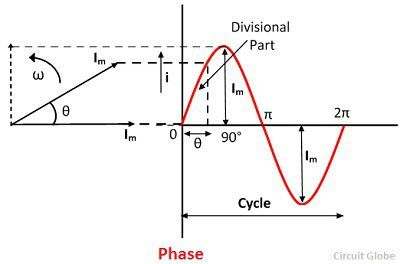# Phase & Phase Difference

## Phase

Definition: The phase of an alternating quantity is defined as the divisional part of a cycle through which the quantity moves forward from a selected origin. When the two quantities have the same frequency, and their maximum and minimum point achieve at the same point, then the quantities are said to have in the same phase.Consider the two alternating currents Im1 and Im2 shown in the figure below. Both the quantity attains their maximum and minimum peak point at the same time. And the zero value of both the quantities establishes at the same instant.

##Phase Difference

Definition: The phase difference between the two electrical quantities is defined as the angular phase difference between the maximum possible value of the two alternating quantities having the same frequency.

In other words, the two alternating quantities have phase difference when they have the same frequency, but they attain their zero value at the different instant. The angle between zero points of two alternating quantities is called angle of phase differences.

Consider the two alternating currents of magnitudes Im1 and Im2 are shown vectorially.Both the vector is rotating at the same angular velocity of ω radians per seconds. The two current obtains the zero value at different instants. Therefore, they are said to have the phase difference of angle φ.The quantity which attains its +ve maximum value before the other is called a leading quantity, whereas the quantity which reaches its maximum positive value after the other, is known as a lagging quantity. The current Im1 is leading the current on Im2 or in other words, current Im2 is the lagging current on Im1.

Cycle: When the alternating quantity goes from through a complete set of +ve or –ve value or goes through 360° electrical degrees, then it is said to have completed a cycle completely.